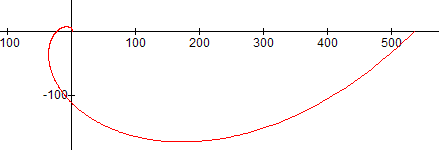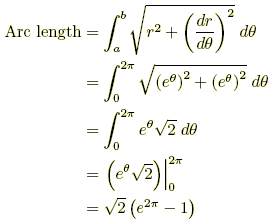# arc length of a curve

Arc Length of a Curve

The length of a curve or line.

The length of an arc can be found by one of the formulas below for any differentiable curve defined by rectangular, polar, or parametricequations.

For the length of a circular arc, see arc of a circle.

 Formula:where a and b represent x, y, t, or θ-values as appropriate, and ds can be found as follows. 1. In rectangular form, use whichever of the following is easier: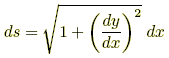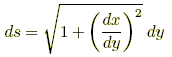2. In parametric form, use3. In polar form, useExample 1: Rectangular Find the length of an arc of the curvey = (1/6) x3 + (1/2) x–1 from x = 1 to x = 2.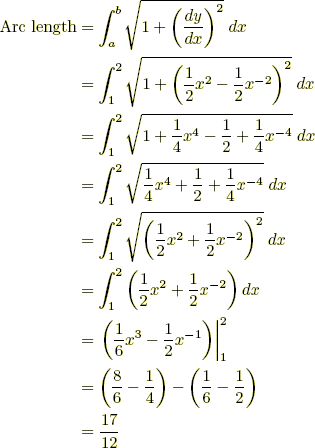Example 2: Parametric Find the length of the arc in one period of the cycloidx = t – sin t,y = 1 – cos t. The values of t run from 0 to 2π.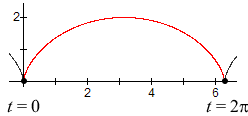Example 3: Polar Find the length of the first rotation of the logarithmic spiralr = eθ. The values of θ run from 0 to 2π.